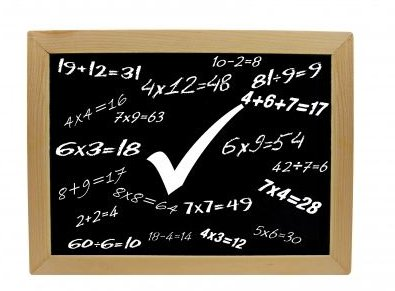# Math: An Absolute Values and Integers Quiz for Students

## Integer Overview

As you work on learning absolute value and integers, take notes on the material– it becomes a vital part of a quiz.

“Absolute value” of a number is its placement away from zero on a number line. The number three is three places away from zero, making its absolute value three. In the same way, the number -3 is three places away from zero. However, instead of being expressed as -3, it is expressed as a positive. You can force a number to become a negative when you place a minus sign in front of it. When you do this, you negate a positive number. Look at some numbers: , , [-5], , [-1]. By adding brackets around each number, you read it as “the absolute value of [X].”

Equations are written out as:  = X, making X = 8. Another sample could be [X] = 2, making this X = 2 or X = -2. If you have an equation written as a negative, there is no solution– the absolute value must be positive. When an absolute value has regular numbers, also called “constants,” it is easier to work with them.## Tips for Taking the Quiz

Bring the number line back out as you work on the different operations affecting positive and negative integers. Beginning with addition, a positive integer is added when you move over to the right on the number line. Add: 1 + 3 = 4. Add a negative integer by moving in the opposite direction–to the left–on the number line. Add two negative integers: -5 + -2 = -7.

Add integers with different signs by keeping the sign of the number with the largest absolute value, and then subtract the smallest absolute value from the largest number: -8 + 2 = -6. Add a negative integer to a positive: 3 + -7 = -4. In this operation, you keep the sign from the integer with the largest absolute value, and then subtract the smallest absolute value from the largest. Keep the negative sign (-4). Subtract an integer by adding its opposite: 4 – 6 = -2. Subtract two negative integers: -5 – [-8] = 13. To subtract two negative integers, add their opposite.

Multiply two positive integers and get a positive result: 4 x 4 = 16. When you multiply a negative with a negative, your answer will be a positive: [-8] x [-7] = 56. When you multiply a positive times a negative, your answer will be a negative:  x [-6] = -54. Finally, when you multiply a negative times a positive, your answer will, again, be a negative: [-8] x  = -64. Take this a little further: If your equation has an odd number of negatives, the final answer is a negative; if your equation has an even number of negative signs, the answer must be a positive. [-3] x [-2] x [-5] = -30; [-3] x  x [-5] = 30.

Dividing positive and negative integers works in the same way as if you are multiplying. [-4] /  = -2.  / [-3] = -5. [-15] / [-5] = 3.

## Absolute Values and Integers Quiz

Opps, Quiz engine needs updating…

## References

• Photo Credit:

Math Symbols Blackboard by Grant Cochrane on FDP

• Free Math Help, Absolute Value, http://www.freemathhelp.com/absolute-value.html

Math.com, Adding and Subtracting Integers, http://www.math.com/school/subject1/lessons/S1U1L11GL.html

Learning Wave, Multiplying Integers, http://www.learningwave.com/chapters/integers/mlt.html

Math Guide, Operations on Integers, http://www.mathguide.com/lessons/Integers.html